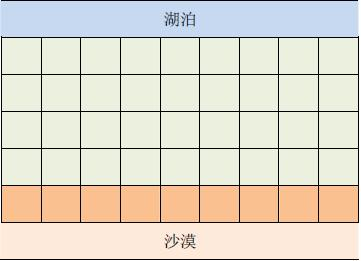# 引子：# 题目描述：# 输入1：

2 5
9 1 5 4 3
8 7 6 1 2


# 输出1：

1
1


# 输入2：

3 6
8 4 5 6 4 4
7 3 4 3 3 3
3 2 2 1 1 2


# 输出2：

1
3


# 代码：

#include<iostream>
#include<cstring>
#include<cstdio>
#include<cstdlib>
#include<cmath>
#include<algorithm>
using namespace std;
int x=0,f=1;char ch=getchar();
while(ch<'0'||ch>'9'){
if(ch=='-')f=-1;
ch=getchar();}
while(ch>='0'&&ch<='9'){
x=x*10+ch-'0';
ch=getchar();}
return x*f;
}
int xx={1,-1,0,0},yy={0,0,1,-1};
int n,m;
int a,mp,l,r;
int qx,qy;
struct data{int l,r;}c;
bool operator<(data a,data b){
return a.l==b.l?a.r<b.r:a.l<b.l;//运算符重载，改为 >
}
void bfs(int b,int x,int y,int v,bool f){
qx=x;qy=y;b[x][y]=v;//初始化
for(int k=0;k<4;k++){//开始搜索
int nowx=x+xx[k],nowy=y+yy[k];
if(nowx<1||nowy<1||nowx>n||nowy>m||b[nowx][nowy])continue;//多方向扩展搜索矩阵图时考虑跳出矩阵情况
if(f==0&&a[nowx][nowy]>=a[x][y])continue;//海拔比原点低，则不连通
if(f==1&&a[nowx][nowy]<=a[x][y])continue;
b[nowx][nowy]=b[x][y];
qx[tail]=nowx;qy[tail]=nowy;tail++;
}
}
}
int main(){
for(int i=1;i<=n;i++)
for(int j=1;j<=m;j++)
for(int i=1;i<=m;i++)
bfs(mp,1,i,1,0);
int tot=0;
for(int i=1;i<=m;i++)
if(!mp[n][i])tot++;
if(tot)	{
printf("0\n%d\n",tot);//有不连通的直接结束
return 0;
}
for(int i=1;i<=m;i++)if(!l[n][i])bfs(l,n,i,i,1);//建了输水站就不可以建蓄水站
for(int i=m;i;i--)if(!r[n][i])bfs(r,n,i,i,1);
for(int i=1;i<=m;i++)	{
if(!l[i])l[i]=r[i];
if(!r[i])r[i]=l[i];
c[i].l=l[i];c[i].r=r[i];
}
int now=0,to=0;
sort(c+1,c+m+1);//否则贪心排序
for(int i=1;i<=m;i++)	{
if(now+1>=c[i].l)to=max(to,c[i].r);
else now=to,to=max(to,c[i].r),tot++;//区间连续所以可以这样找
}
if(now!=m)tot++;
printf("1\n%d\n",tot);
return 0;
}

09-2761007-27615
09-21472
08-162143
10-26192
03-1489
08-01
10-215079